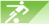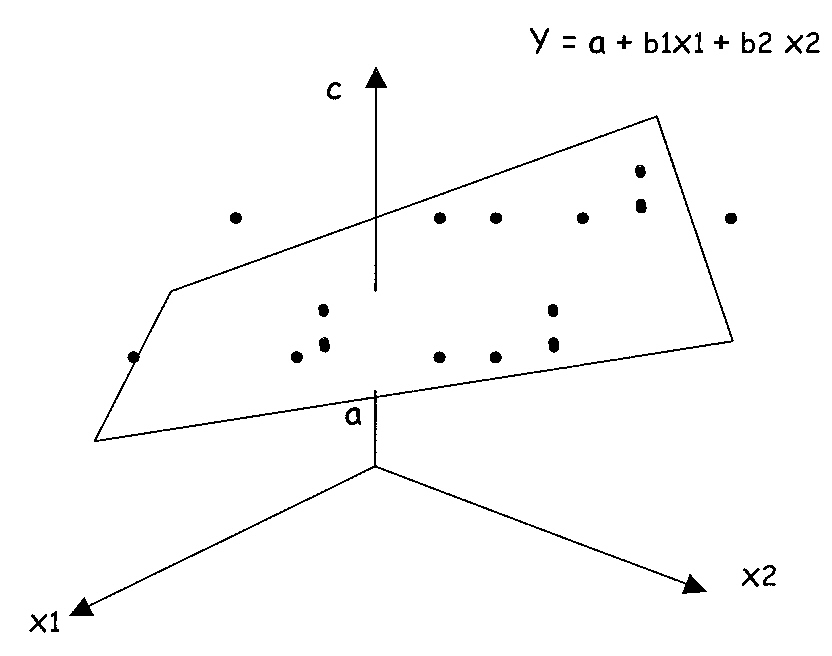Understanding RealTimeRPI GAMER 1. What is GAMER? GAMER is a comprehensive sports analysis and prediction system developed by RealTimeRPI.com. GAMER is virtually an advanced statistical prediction model, and it's the most accurate sports prediction system available on the Net with 76.8% accuracy. 2 Can you brifely describe the statistical model used by GAMER? GAMER uses a multivariable regression model to simulate sports events. The multivariable regression equation deals with the following variables: The unknown parameters denoted as K; This may be a scalar or a vector of length n. The independent variables, X. The dependent variable, Y. The multivariable Regression equation can be described as a function of variables X and K: Y = f (X, K ); Take an example, in a simple two variable regression model, we can use following figure to fit a regression plane to a set of samples in 2-Dimension space:We assume that c is a function of several inputs x1, x2,...xn (independent variables), and the goal is to find the best regressor of c on all the inputs (Figure above). For Dimemsion = 2 this corresponds to fitting a plane through the N input samples or a hyperplane in the general case of N dimensions. 3. What variables go into GAMER? Most important variables considered in GAMER are listed as follows: A team's winning percentage A team's strength of schedule (SOS). Home court advantages Oppenents' strength of schedule A team's scores Opponents' scores Winning margins, 4. How accurate is your GAMER prediction system? In general, GAMER performs well in our research. Our back-testing shows a remarkable 76.8% overall accuracy. Early season results are slightly lower because of the data availability issue.